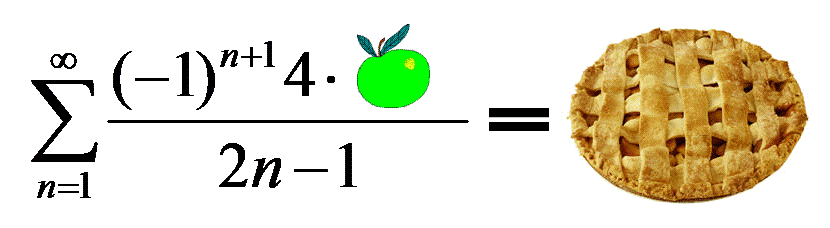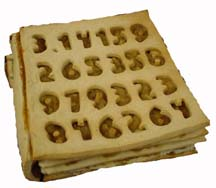# Resolutions

Okay, okay, okay.   I suppose I ought to resolve all the paradoxes I posed a while back.   I’ve actually been delaying this, since I posed the second paradox to my summer school algebra students to see if they could figure it out, since a reasonable explanation can be made using the concept of “slope,” which we’ve just covered.

So let’s do it!

## Paradox the first

The first paradox involved a triangle that could be dissected and reassembled into  a proper subset of itself.

The flaw is in calling it a triangle — it’s not.   It’s actually two different shapes.   Examining the first shape, the slope of the “red” portion of the “hypotenuse” is 3/8 — a 37.5o grade.   The blue portion, however, has a slope of 2/5 — a 40o grade.   Said differently, the “hypotenuse” of the “triangle” is actually ever-so-slightly concave, so the shape isn’t a triangle at all — it’s a quadrilateral.   Moreover, that slight difference from the true diagonal is exactly 1/2 of a square-unit of area.   When the shape is reassembled, the new “diagonal” is now slightly convex, pushing outward another extra 1/2 square-unit of area — and thus leaving room for exactly one square unit of area to be displaced.

This paradox was discovered in 1953 by Paul Curry, an amateur magician from New York City.   Truly astute eyes will observe that 3, 5, and 8 are consecutive Fibonacci numbers.   In fact, any three consecutive Fibonacci numbers can be used to make a “triangle’s missing square” dissection — experiment for yourself!

## Paradox the second

The second paradox involved a square that could be dissected and reassembled into a rectangle with a larger area.

The second paradox is merely a variation on the first: the “diagonal” of the rectangle isn’t.   Instead, both edges are  slightly concave, leaving a very long, very skinny parallelogram going from corner to corner within the triangle.   It’s area? You guessed it: exactly 1 square unit.

As with the previous paradox, notice that all the pieces are of lengths 3, 5, 8, and 13 — consecutive Fibonacci numbers again.   And as with the previous paradox, this dissection can be remade using any 4 consecutive Fibonacci numbers, as illustrated with this applet.

## Paradox the third

The third paradox involved a group of 12 people who could be dissected and rearranged into 13 people.

It’s actually a variation on a Sam Loyd puzzle called Disappearing leprechauns.   Once again, the good folks at Cut-the-knot have a nice applet which shows this illusion in a very simplified form: a collection of n rectangles which can be (occasionally) rearranged into n-1 rectangles.Perhaps the easiest way to explain the paradox is that each of the initial people is cut into  two pieces, starting at the head and moving down exactly 1/12 the height from person to person.   Each slice is then moved to the “next” person, overlapping a 1/12th portion — except for the guy who lost  his bottom 1/12th (number  13 above).   The result is thirteen people, each of whom is only 11/12ths his original height.    (The  order of the people is mucked about  to hide this fact.)

The solution image above, by the way, comes from Matthew Baldwin over at defective yeti, who offers an excellent and in-depth discussion of the 12 man/13 man paradox.

## Paradox the fourth

The missing dollar paradox really isn’t a paradox at all — it’s just a case of misdirection. If you want to, just re-enact the situation with Monopoly money, and you’ll see that all of the original 30 dollars spent can be easily accounted for: 25 of them are in the manager’s cash register, 3 of them are back with the mathematicians, and 2 of them are with the bellboy.

The misdirection is in asking to account for the 30 dollars paid.   Reread the story — the mathematicians didn’t pay for a \$30 room — they actually paid for a \$25 room.   Having each paid 10 dollars and getting one dollar back, the mathematicians have, in effect, paid \$27 for a \$25 dollar room, so the actual question to ask is: What happened to the two extra dollars?

…and this is far less mysterious.

## Finally…

The answer to the summer summation:As proof, recall from calculus that the Taylor series expansion of the arctangent function is given by$displaystyle arctan(x) = x - frac{x^3}{3} + frac{x^5}{5} - frac{x^7}{7} + frac{x^9}{9} - cdots$

This series converges on the closed interval [-1,1].   Substituting$x = 1$ into this equation yields a series summation for arctan(1), which is$pi/4$; rearranging yields$displaystyle pi = 4 bigg( 1 - frac{1}{3} + frac{1}{5} - frac{1}{7} + frac{1}{9} - cdots bigg)$

which is known as Gregory’s series.   Multiplying both sides an apple yields the result: apple$pi$.*

* This is the way a mathematician makes a bakery joke.   This, on the other hand, is how a baker makes a makes a mathematical joke:This entry was posted in nerdify. Bookmark the permalink.